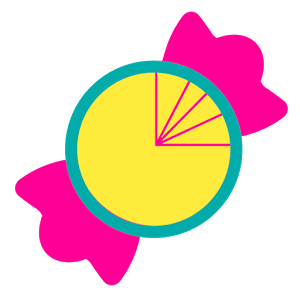\$0.99

# Android Giveaway of the Day - Trig or Treat

\$0.99 期限切れ
ユーザーの評価: 1 (100%) 0 (0%)

このgiveawayオファーはすでに期限が切れています。Trig or Treatが今紹介されています。

*** Ace your next trigonometry test! ***

This fun trigonometry game is divided into three modules:

1. Unit Circle Angles - a) Guess the correct angle given the coordinates on the unit circle
b) Guess the correct coordinates on the unit circle given the angle

2. Trig Values - Find the correct trigonometric value given the angle (you decide the unit for the angle: radians, degrees, or a mix of both)

3. Trig Inverse Values - Find the correct inverse trigonometric value given the angle (you decide the unit for the angle: radians, degrees, or a mix of both)

For every correct answer you receive one candy, and for every incorrect answer you lose one candy. The goal is to get 10 candies in total. The trigonometry game ends when you have 10 candies or if you run out of candies.

### 開発者:

Math Training Apps

Educational

2.0

Everyone

4.0.3 and up

## コメント Trig or Treat

Thank you for voting!